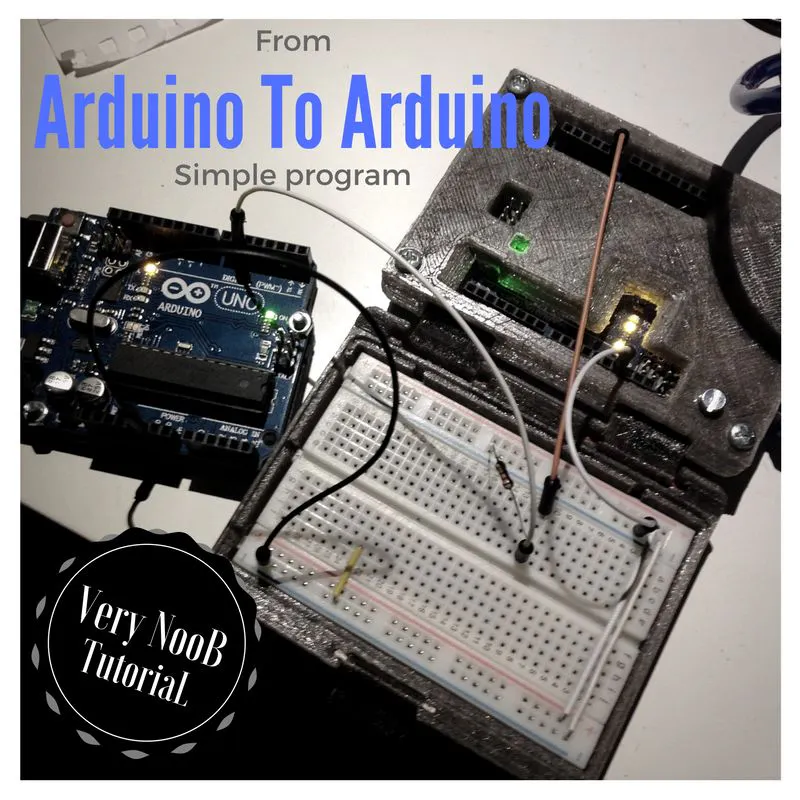Communication Between Arduino UNO © GPL3+

If you want use two Arduino, you can use a simple I/O port to communicate. The point of question is the Ground.

• 4,167 views
• 1 comment
• 7 respects

Apps and online services

If you want use two Arduino, you can use a simple I/O port to communicate.

The point of question is the Ground. When you connect two Arduino with each other, you have to connect their ground. In this tutorial when the Arduino UNO number 1 lights ON the onboard LED, the Arduino UNO number 2 connects to the computer, prints HIGH on the serial port and switches ON the onboard LED.

The result is two Arduino UNO that blink together.

Step 1: The connections of Arduino UNO to Arduino UNO

See the image and connect two Arduino.

Step 2: Now the code

Now download two files. Upload the sketch Output on Arduino UNO number 1 and Input sketch on Arduino UNO number 2. Now connect the Arduino UNO number 2 to the computer and open the Serial port to 9600 baud.

You can see the LED on board of Arduino UNO number 1 blinking, and at same time you can see the LED on board of Arduino UNO number 2. If you disconnect pin number 6 of the Arduino UNO number 1 from the breadboard, you can see the blinking only on the Arduino UNO number 1.

Code

Arduino UNO AArduino
#define LED 13
#define IN A0
int val = 0;

void setup() {
// put your setup code here, to run once:
Serial.begin(9600);
pinMode(IN, INPUT);
pinMode(LED, OUTPUT);
}

void loop() {
// put your main code here, to run repeatedly:
Serial.println(val);
if (val >= 740) {
digitalWrite(LED, HIGH);
Serial.println("HIGH");
}
else {
digitalWrite(LED, LOW);
}
//delay(50);
}
Arduino UNO BArduino
#define SMS 6
#define LED 13

void setup() {
pinMode(SMS, OUTPUT);
pinMode (LED, OUTPUT);
}

void loop() {
digitalWrite(SMS, HIGH);
digitalWrite(LED,HIGH);
delay(2000);
digitalWrite(SMS, LOW);
digitalWrite(LED, LOW);
delay(200);
}

Multicolor Knight Rider with RGB PL9823 LED + Arduino UNO

Project tutorial by Giovanni Gentile

• 3,925 views
• 10 respects

• 23,718 views
• 14 respects

• 10,151 views
• 5 respects

Arduino UNO MQTT Meteo Station

Project tutorial by Giovanni Gentile

• 5,008 views
• 4 respects

• 3,601 views